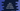# Java program to subtract one matrix from another### Java program to subtract one matrix from another :

In this Java programming tutorial, we will learn how to subtract one matrix from another matrix. The program will ask the user to enter values for both of the matrices and it will calculate the subtraction result. Finally, the program will print out the result matrix.

### How to calculate the subtraction :

To calculate the final subtraction result or the final matrix, we will have to subtract each value of the second matrix from the first matrix. The values are of the same row and column number for both matrices. Means, if the first matrix is A and the second matrix is B, we will do the subtraction A[i][j] - B[i][j] for all values of row i and column j.

Let’s take a look at the Java program first :

### Example Program :

``````import java.util.Scanner;

public class Main {

//1
/**
* Utility functions for System.out.println() and System.out.print()
*/
private static void print(String str) {
System.out.print(str);
}

private static void println(String str) {
System.out.println(str);
}

//2
/**
* Function to print a matrix
* @param matrix : Matrix to print
* @param row : row count of the matrix
* @param column : column count of the matrix
*/
private static void printMatrix(int[][] matrix, int row, int column) {
for (int i = 0; i < row; i++) {
for (int j = 0; j < column; j++) {
print(matrix[i][j] + " ");
}
println("");
}
}

//3
/**
* Get inputs for a matrix
* @param matrix : inputs will be save in this matrix
* @param row : row count for the matrix
* @param column : column count of the matrix
*/
private static void getInputsForMatrix(int[][] matrix, int row, int column) {
Scanner scanner = new Scanner(System.in);

for (int i = 0; i < row; i++) {
for (int j = 0; j < column; j++) {
matrix[i][j] = scanner.nextInt();
}
}

}

//4
/**
* Subtract one matrix from another
* @param matrix1 : First matrix
* @param matrix2 : Second matrix
* @param row : row count of both the matrix
* @param column : column count of both the matrix
* @return : matrix1 - matrix2
*/
private static int[][] subtract(int[][] matrix1, int[][] matrix2, int row, int column) {
int[][] resultMatrix = new int[row][column];

for (int i = 0; i < row; i++) {
for (int j = 0; j < column; j++) {
resultMatrix[i][j] = matrix1[i][j] - matrix2[i][j];
}
}

return resultMatrix;
}

public static void main(String args[]) {
//5
int row;
int column;

//6
Scanner scanner = new Scanner(System.in);

//7
//get row count
println("Enter row of both matrix : ");
row = scanner.nextInt();

//8
//get column count
println("Enter column of both matrix : ");
column = scanner.nextInt();

//9
int[][] matrix1 = new int[row][column];
int[][] matrix2 = new int[row][column];

//10
//get inputs for both matrix
println("Enter the First Matrix :");
getInputsForMatrix(matrix1, row, column);

println("Enter the second Matrix :");
getInputsForMatrix(matrix2, row, column);

//11
//calculate the result
int[][] resultMatrix = subtract(matrix1, matrix2, row, column);

//12
print("First matrix - Second Matrix : ");

//print result matrix
printMatrix(resultMatrix, row, column);

}
}
``````

### Explanation :

The commented numbers in the above program denote the step numbers below :

1. print and println functions are utility functions. We have created these functions so that we don’t need to write System.out.println and System.out.print again and again.

2. printMatrix function takes one matrix and its row and column count as arguments and prints out the matrix to the user.

3. getInputsForMatrix function takes one matrix, the row count and column count as input, reads the inputs from the user and put it in the matrix.

4. subtract function takes two matrices as arguments and also it takes the row and column count as input. Then it finds out the subtraction of the matrix and returns the final result.

5. The main() function will run first. Create two integer variables row and column to store the row and column values of both matrices. The size of the matrix should be the same to calculate the subtraction.

6. Create one Scanner object to read the user input values.

7. Read the row count and store it in the row variable.

8. Read the column count and store it in the column variable.

9. Create two matrices with the same row and column count as inserted by the user.

10. Get input from the user for both of the matrix.

11. Calculate the subtraction of the two matrix and store it in resultMatrix variable.

12. Print out the final subtraction result.

### Example Output :

``````Enter row of both matrix :
3
Enter column of both matrix :
3
Enter the First Matrix :
9 7 6
8 8 8
7 9 3
Enter the second Matrix :
8 3 1
7 7 7
1 1 1
First matrix - Second Matrix :
1 4 5
1 1 1
6 8 2
``````